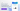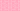Pricing# How to Calculate Marginal Cost

Written by

It’s essential to have a strong understanding of marginal costs if you want to maximize your profits and decrease the cost-per-unit of production. Find out everything you need to know about how to calculate marginal cost.

We’ll explore the marginal cost formula, take you through an example of a marginal cost equation, and explain the importance of marginal costs for business in a little more depth.

## What is marginal cost?

Marginal cost is a term used in economics and accounting that refers to the incremental costs involved in producing additional units. In any marginal cost equation, you’ll need to include the variable costs of production. For example, labor and materials will need to be considered. However, you’ll only need to include the fixed costs of production (i.e., administrative costs, overheads, sales expenses, etc.) if there’s a need to increase your fixed costs to handle the additional output.

## Marginal cost formula

If you want to learn how to calculate marginal cost, you can use the following marginal cost formula:

Marginal Cost = Change in Total Cost / Change in Quantity

Let’s explore the two main elements of the marginal cost formula in a little more depth:

1. Change in Total Cost – At any level of production, your costs can increase or decrease. If you need to hire an extra worker or purchase more raw materials to make additional units, for example, your production costs will increase. To find out how much your production costs have changed, you can deduct the production cost of batch one from the production cost of batch two.

2. Change in Quantity – Of course, volumes will also increase or decrease whenever you have differing levels of production. To work out the change to your quantities, you’ll need to deduct the number of goods from your first production run from the number of goods from the second, expanded production run.

## Example of marginal cost

Using the marginal cost formula, let’s explore how marginal cost works in the real world with an example. Imagine that Company A regularly produces 10 handcrafted tables at the cost of \$2,000. However, demand spikes and they receive more orders, leading them to purchase more materials and hire more employees. In their next production run, they produce 20 units at the cost of \$3,000.

Marginal Cost = (\$3,000 – \$2,000) / (20 – 10) = \$100

In other words, the marginal cost (i.e., the additional expenditure to make another unit) is \$100 per table.

## Why is the marginal cost equation important?

Knowing how to calculate marginal costs is vital for a couple of reasons. Most importantly, it provides you with an insight into the efficiency of your production schedule, giving you a way to determine at what point your company will be able to achieve economies of scale (i.e., cost efficiencies resulting in a decreased cost-per-unit). The quicker you can reach an optimum production level, the better for your business. Put simply, if the marginal cost of producing one additional unit is lower than the purchase price, the company can make a profit.

It’s also important to note that your business’s marginal cost curve may begin to increase if your company becomes less productive and suffers from diseconomies of scale (i.e., the inverse of an economy of scale, wherein the business becomes too large, and poor communication, loss of control, and external opposition lead to a rise in per-unit costs). If the amount of revenue you’re generating (marginal revenue) is the same – or less – than the marginal cost, you’ll need to call a halt to production, as the cost of production is causing the business to lose money.

## We can help

GoCardless helps you automate payment collection, cutting down on the amount of admin your team needs to deal with when chasing invoices. Find out how GoCardless can help you with ad hoc payments or recurring payments.